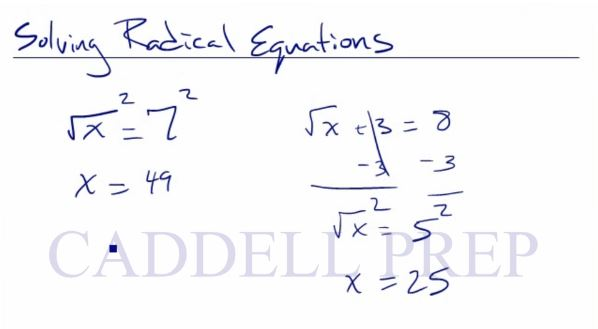## Part 1

In this video, we are going to solve equations with a radical expression. After you finish this lesson, view all of our Algebra 1 lessons and practice problems.

In$\sqrt{x}=7$, first square both sides of the equation$\sqrt{x}^2=7^2$$x=49$

If there are other values in the equation, then first isolate the$\sqrt{x}$ before squaring both sides of the equation.

## Video-Lesson Transcript

Let’s go over how to solve equations with a radical expression.

We have$\sqrt{x} = 7$

In order to solve for$x$, we have to get rid of the radical sign.

So we’ll square both sides$(\sqrt{x})^2 = 7^2$$x = 49$

If we have something like this:$\sqrt{x} + 3 = 8$

we want to get the radical by itself first.

Let’s subtract$3$ on both sides$\sqrt{x} + 3 - 3 = 8 - 3$$\sqrt{x} = 5$

And the last step is to square both sides.$(\sqrt{x})^2 = 5^2$$x = 25$Let’s look at a more complicated one$3 \sqrt{x} - 4 = 7$

Again, we want to have the radical by itself. Let’s add$4$ on both sides.$3 \sqrt{x} - 4 + 4 = 7 + 4$$3 \sqrt{x} = 11$$\dfrac{3 \sqrt{x}}{3} = \dfrac{11}{3}$$\sqrt{x} = \dfrac{11}{3}$$(\sqrt{x})^2 = (\dfrac{11}{3})^2$$x = \dfrac{121}{9}$

Let’s have this:$\sqrt{x - 8} = 3$

Here, the whole term is under the radical, so it is already isolated.

So, we’ll start by squaring both sides.$(\sqrt{x - 8})^2 = 3^2$$x - 8 = 9$

Now, let’s solve for$x$.$x - 8 + 8 = 9 + 8$$x = 17$

## Part 2

In the second part, we are going to solve equations with a radical expression that are more complex.

For example:$\sqrt{x}=(x-5)$

Square both sides of the equation$\sqrt{x}^2=(x-5)^2$

Use FOIL to complete the squaring if necessary$x=(x-5)(x-5)$$x=x^2-5x-5x+25$

Combine like terms$x=x^2-10x+25$$x=\frac{-b\pm\sqrt{b^2-4ac}}{2a}$

Substitute the variables$x=\frac{11\pm\sqrt{(-11)^2-4(1)(25)}}{2(1)}$$x=\frac{11\pm\sqrt{121-4(1)(25)}}{2(1)}$$x=\frac{11\pm\sqrt{21}}{2}$

So we have:$x=\frac{11+\sqrt{21}}{2}$ and$x=\frac{11-\sqrt{21}}{2}$

In the end, x is approximately 7.8 or 3.2

If it was -7.8 instead of 7.8, then it cannot be a negative answer since the square root of a negative number is imaginary. In this case, the final answer would just be 3.2.## Examples of Solving Equations That Have Radical Terms

### Example 1$\sqrt{2x-2}=x-1$

First, square both sides of the equation to eliminate the radical symbol$(\sqrt{2x-2})^2=(x-1)^2$
Then, solve the equation that comes out after the squaring process$2x-2=(x-1)(x-1)$$2x-2=x^2-2x+1$

Get all the terms on one side so the other side be zero.$2x-2=x^2-2x+1$$2x-2+2=x^2-2x+1+2$$2x=x^2-2x+3$$2x-2x=x^2-2x+3-2x$$0=x^2-4x+3$
Now, we can factor out the quadratic function$0=(x-1)(x-3)$
Let’s solve for$x$$x-1=0$$x=1$
and$x-3=0$$x=3$$(1, 3)$

### Example 2$\sqrt{5x+11}-1=x$
First, add -1 from both sides$\sqrt{5x+11}-1+1=x+1$$\sqrt{5x+11}=x+1$
Now, we square both sides of the equation to eliminate the radical symbol$(\sqrt{5x+11})^2=(x+1)^2$
Then, solve the equation that comes out after the squaring process$5x+11=(x+1)(x+1)$$5x+11=x^2+2x+1$

Get all the terms on one side so the other side be zero.$5x+11-11=x^2+2x+1-11$$5x=x^2+2x-10$$5x-5x=x^2+2x-10-5x$$0=x^2-3x-10$
Now, we can factor out the quadratic function$0=(x+2)(x-5)$
Let’s solve for$x$$x+2=0$$x=-2$
and$x-5=0$$x=5$
Remember: if one of the answers come out as a negative, that’s a rejected answer.
Therefore, our final answer is$5$

## Video-Lesson Transcript

This is how to solve equations with a radical expression part 2.$\sqrt{x} = x - 5$

It’s a little bit different because we have$x$ on both sides.

Here, we want to get the radical by itself first before we combine the$x$ together.

In order to get rid of the radical, let’s square both sides.$(\sqrt{x})^2 = (x - 5)^2$$x = (x - 5) (x - 5)$$x = x^2 - 5x - 5x + 25$$x = x^2 - 10x + 25$

Now, we have a quadratic equation.

We want to get all the terms on one side so the other side be zero.$x - x = x^2 - 10x - x + 25$$0 = x^2 - 11x + 25$

This can not be factored so let’s just use the quadratic function to solve it.

Our formula is$x = \dfrac{-b \pm \sqrt{b^2 - 4ac}}{2a}$

Here we have$a = 1, b = -11, c = 25$$x = \dfrac{11 \pm \sqrt{121 - 4(1)(25)}}{2 (1)}$$x = \dfrac{11 \pm \sqrt{21}}{2}$

{$\dfrac{11 + \sqrt{21}}{2}, \dfrac{11 - \sqrt{21}}{2}$}

What is important here is that we always want to make sure that we won’t end up with a negative radical.

Let’s find out what is the exact value of this is$\sqrt{21} = 4.6$
{$\dfrac{11 + 4.6}{2} = 7.8$}
{$\dfrac{11 - 4.6}{2} = 3.2$}

If one of the answers come out as a negative, that’s a rejected answer.

The only answer we accept is a positive number. Because you cannot find the square root of a negative number.

So, you always have to check and make sure that you don’t have a negative number under the radical. After you finish this lesson, view all of our Algebra 1 lessons and practice problems.